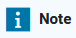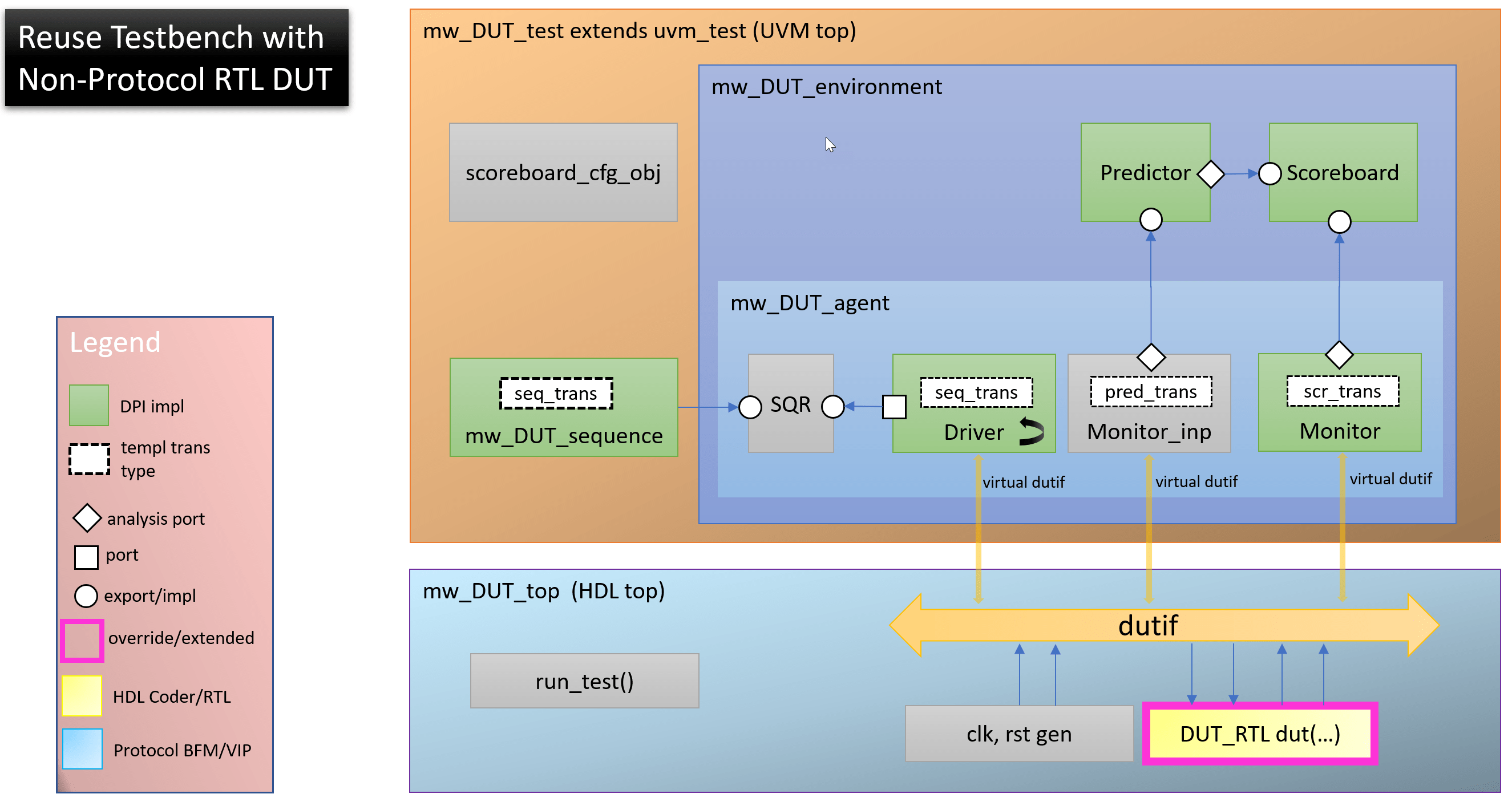# Replace Behavioral DUT with AXI-Based RTL DUT in UVM Test Bench

This example shows how to move from a simple behavioral DUT interface to an RTL DUT interface which uses AXI Lite and AXI Stream bus protocols. Several of the UVM components must change such as the driver and monitor, but the original test bench structure and the sequence and scoreboard components can be re-used without modification.

### Introduction

See the example `Generate Parameterized UVM Test Bench from Simulink` for a description of the design and the background on generating a UVM test bench. To generate the default test bench for this example execute:

```% Generate a UVM test bench design = 'prm_uvmtb/PulseDetector' sequence = 'prm_uvmtb/GenPulse' scoreboard = 'prm_uvmtb/CheckDetection' uvmbuild(design, sequence, scoreboard) ```

The DUT in Simulink represents the functional behavior of the pulse detector and does not use any hardware protocol interfaces that are typical of HDL IP cores. The UVM test bench uses the Simulink design as a stand-in for the actual HDL implementation. The next step in the verification workflow integrates an actual HDL implementation that uses AXI-based protocols into the same generated UVM test bench.

To use an RTL DUT you must substitute pieces of the UVM test bench, as shown in blue:### Mapping Ports to AXI Interfaces

For this RTL DUT, the `coeff` port is mapped to a processor interface, AXI4-Lite, the `data_in` port is mapped to an AXI4-Stream slave interface, and the `data_out` port is mapped to an AXI4-Stream master interface as shown below.You can manually write this RTL or use HDL Coder's IP core generation workflow to create it. This model has a variant algorithm which can generate an HDL IP core using the HDL Coder product.

### Simulating the HDL Variant in Simulink

To simulate with this variant execute:

```% Simulate the RTL generatable algorithm variant set_param('prm_uvmtb/PulseDetector','LabelModeActiveChoice','HDL') set_param('prm_uvmtb/CheckDetection/ExpectsDelay','LabelModeActiveChoice','Frame delay') sim('prm_uvmtb') ``````[FrameNum= 0] No peak found in Ref or Impl. [FrameNum= 1] Peak location=2163.000000, mag-squared=0.280 using global max [FrameNum= 1] Peak detected from impl=2170 error(abs)=7 [FrameNum= 1] Peak mag-squared from impl=0.280, error(abs)=0.000 error(pct)=0.017 [FrameNum= 2] Peak location=2163.000000, mag-squared=0.200 using global max [FrameNum= 2] Peak detected from impl=2170 error(abs)=7 [FrameNum= 2] Peak mag-squared from impl=0.199, error(abs)=0.000 error(pct)=0.190 [FrameNum= 3] Peak location=2163.000000, mag-squared=0.224 using global max [FrameNum= 3] Peak detected from impl=2170 error(abs)=7 [FrameNum= 3] Peak mag-squared from impl=0.223, error(abs)=0.000 error(pct)=0.183 [FrameNum= 4] Peak location=2163.000000, mag-squared=0.200 using global max [FrameNum= 4] Peak detected from impl=2170 error(abs)=7 [FrameNum= 4] Peak mag-squared from impl=0.200, error(abs)=0.000 error(pct)=0.043 [FrameNum= 5] Peak location=2163.000000, mag-squared=0.255 using global max [FrameNum= 5] Peak detected from impl=2170 error(abs)=7 [FrameNum= 5] Peak mag-squared from impl=0.255, error(abs)=0.000 error(pct)=0.031 [FrameNum= 6] Peak location=2163.000000, mag-squared=0.241 using global max [FrameNum= 6] Peak detected from impl=2170 error(abs)=7 [FrameNum= 6] Peak mag-squared from impl=0.241, error(abs)=0.000 error(pct)=0.187 [FrameNum= 7] Peak location=2163.000000, mag-squared=0.241 using global max [FrameNum= 7] Peak detected from impl=2170 error(abs)=7 [FrameNum= 7] Peak mag-squared from impl=0.241, error(abs)=0.000 error(pct)=0.019 [FrameNum= 8] Peak location=2163.000000, mag-squared=0.225 using global max [FrameNum= 8] Peak detected from impl=2170 error(abs)=7 [FrameNum= 8] Peak mag-squared from impl=0.225, error(abs)=0.000 error(pct)=0.032 [FrameNum= 9] Peak location=2163.000000, mag-squared=0.239 using global max [FrameNum= 9] Peak detected from impl=2170 error(abs)=7 [FrameNum= 9] Peak mag-squared from impl=0.239, error(abs)=0.001 error(pct)=0.241 [FrameNum= 10] Peak location=2163.000000, mag-squared=0.225 using global max [FrameNum= 10] Peak detected from impl=2170 error(abs)=7 [FrameNum= 10] Peak mag-squared from impl=0.225, error(abs)=0.000 error(pct)=0.146 ```### Generating the HDL

If you have HDL Coder, you can set up a tool path and generate RTL for this variant. For example, to create Xilinx Vivado compatible IP, you plug in your tool path and execute the following:

```% Generate the AXI-based RTL HDL IP (HDL Coder required) hdlsetuptoolpath('ToolName', 'Xilinx Vivado', 'ToolPath', '/tools/Vivado/2018.3/bin/vivado') prm_uvmtb_hdlworkflow ```

This will place the resulting HDL IP files into hdl_prj/hdlsrc/prm_uvmtb. However, the RTL implementation has been included with this demo located in overrides_AXIDUT/AXIIPSource and so no HDL Coder license is needed.

### Overriding the Necessary UVM Components

The UVM files to replace the original DUT are located in overrides_AXIDUT. There is no need to modify any of the generated files from the original `uvmbuild` invocation.

### Simulating the UVM Test Bench

Execute the test bench with the new RTL DUT to verify the UVM execution matches the Simulink execution. Because the sequence is parameterized with the SNR input port, its default value will be 0.0 in UVM. In order to properly compare the simulation runs, we need to change its default value to 2.0 (which has a bit value of 0b10_000000), to match Simulink; this can be done via a plusarg which we pass to the script via an environment variable.

We have many new RTL files to compile and we must override the top level design unit. We pass these updates to the script through environment variables.

```% Clear environment variables that influence the UVM simulation setenv EXTRA_UVM_SIM_ARGS; setenv EXTRA_UVM_COMP_ARGS; setenv UVM_TOP_MODULE; % Simulate the UVM test bench using the AXI RTL DUT cd prm_uvmtb_uvmbuild/uvm_testbench/top setenv EXTRA_UVM_COMP_ARGS '-f ../../../overrides_AXIDUT/extra_comp_args.f' setenv EXTRA_UVM_SIM_ARGS '+SNR_default_inp_val=10000000 +UVM_TESTNAME=mw_PulseDetector_AXIRTL_test' setenv UVM_TOP_MODULE mw_PulseDetector_AXIRTL_top ! vsim -do run_tb_mq.do % ModelSim/QuestaSim (gui) ! vsim -c -do run_tb_mq.do % ModelSim/QuestaSim (console) ! ./run_tb_incisive.sh % Incisive (console) ! ./run_tb_xcelium.sh % Xcelium (console) ! ./run_tb_vcs.sh % VCS (console) cd ../../.. ```

The simulation log and a waveform snippet are shown below. Notice the log shows the same results as before, 5 detected pulses. However, also notice the waveforms show different timing for exercising the design. Instead of a steady stream of always valid data, for each frame of 5000 signal samples, the 64 coefficients are first programmed via the processor interface, then the 5000 samples are streamed.### Conclusion and Next Steps

This example has shown how a design and test bench developed in Simulink can be used to generate a fully executable UVM test bench. The `uvmbuild` command automates the generation, compilation, and integration of key components into the UVM framework.

HDL verification engineers can confirm overall coverage from Simulink and augment the coverage with their own library of native UVM sequences.

They can also substitute a behavioral design from Simulink with an RTL design that is wrapped in hardware protocols such as AXI4 with no changes to the original sequence generator and response checkers.

## Support